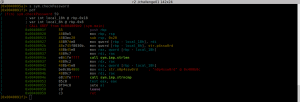# Reverse Engineering With Radare2 – Part 1

Welcome back to the radare2 reversing tutorials. If you’ve missed the intro, you can find it here.

The last time you got the challenge01 binary and your goal was to find the password for the login. Let’s see how the application looks like:

```\$ ./challenge01
##################################
#          Challenge 1           #
#                                #
#      (c) 2016 Timo Schmid      #
##################################
Enter Password: test
Wrong!
```

The first and simplest step would be to look for strings inside the binary. We could do this either by using the unix utility strings or the binary analyzing binary from radare rabin2:

```\$ rabin2 -z ./challenge01
vaddr=0x00400a68 paddr=0x00000a68 ordinal=000 sz=35 len=34 section=.rodata type=ascii string=##################################
vaddr=0x00400a90 paddr=0x00000a90 ordinal=001 sz=35 len=34 section=.rodata type=ascii string=#          Challenge 1           #
vaddr=0x00400ab8 paddr=0x00000ab8 ordinal=002 sz=35 len=34 section=.rodata type=ascii string=#                                #
vaddr=0x00400ae0 paddr=0x00000ae0 ordinal=003 sz=35 len=34 section=.rodata type=ascii string=#      (c) 2016 Timo Schmid      #
vaddr=0x00400b03 paddr=0x00000b03 ordinal=004 sz=9 len=8 section=.rodata type=ascii string=p4ssw0rd
vaddr=0x00400b0c paddr=0x00000b0c ordinal=005 sz=11 len=10 section=.rodata type=ascii string=n0p4ssw0rd
vaddr=0x00400b17 paddr=0x00000b17 ordinal=006 sz=17 len=16 section=.rodata type=ascii string=Enter Password:
vaddr=0x00400b28 paddr=0x00000b28 ordinal=007 sz=6 len=5 section=.rodata type=ascii string=%255s
vaddr=0x00400b2e paddr=0x00000b2e ordinal=008 sz=19 len=18 section=.rodata type=ascii string=Password accepted!
vaddr=0x00400b41 paddr=0x00000b41 ordinal=009 sz=7 len=6 section=.rodata type=ascii string=Wrong!
```

Two strings look interesting: “p4ssw0rd” and “n0p4ssw0rd”. Obviously, we could try both of them if one works, but as this is a reversing tutorial, we’ll figure it out in more depth ????

So after loading the binary into radare (r2 ./challenge01) and do some anlysis (aaa) we’ll start by looking at the main function of the binary. As radare2 seeks to the entry point, we have to seek to the main function first.As we can see in the output above, the main function contains several calls. Some of them are calling (imported) library functions which are named after the scheme sym.imp.<function name>. As this is a x64 binary on a linux system, it is using the System V AMD64 ABI for the calling conventions. This means that the first argument is placed in the rdi register, the second in the rsi, third in rdx, forth rcx, fifth r8 and sixth r9 register. A stack buffer is seemed to also be used as the rsp register is decremented by 0x120, which allocates 288 bytes on the stack. After that, the registers edirsi and rdx are stored on the stack (maybe as backup). After the call to the banner function, a pointer to the string “Enter Password: ” is stored in edi which means in the first argument for the next function call (printf in this case). The next call (scanf) takes the arguments “%255s” and a pointer to the stack. Based on the information shown by radare, the pointer is pointing at position rdp-0x100 which means that it points at a 256 byte buffer on the current stack frame (0x100 = 256 and the last byte is set to zero after the call). Now it become interesting as the pointer to the buffer is given to the checkPassword function as the first argument. The return value of this function (al) is checked if it is zero or not. If it contains zero, the jump at 0x4009c0 will be taken to the address 0x4009ce and “Wrong!” will be printed. Otherwise “Password accepted!”.

Let’s take a look at the checkPassword function:As we can see here, our argument is stored on the stack (at local_18h) after that it is read again to be passed to the strlen function. The result of this function is then used as the third argument to the strncmpfunction. The first argument is our input string and the second argument is …. “n0p4ssw0rd”. This means that the searched password is “n0p4ssw0rd” ???? :

```\$ ./challenge01
##################################
#          Challenge 1           #
#                                #
#      (c) 2016 Timo Schmid      #
##################################
Enter Password: n0p4ssw0rd
Password accepted!
```

Challenge solved!

## Challenge 0x02

You will find the next challenge here (linux64 dynamically linked, linux64 statically linked, win64):

https://github.com/bluec0re/reversing-radare2/tree/master/sources/challenge02

MD5 (challenge02) = 2b26165a67274fca1d23959675114444
MD5 (challenge02.exe) = 5bc1f2451d62ff33b2128185a32cdc9b
MD5 (challenge02-static) = 34ab5bb11a095383c121aecbb689767a
SHA1 (challenge02) = 34c8bb9de8f1c78dbe45edc80c7cedbc176b38a9
SHA1 (challenge02.exe) = 07522e7e3c6444bd3dfae1061d442551b23c6ae6
SHA1 (challenge02-static) = 99d0eac08903ff3d7404d4edb3ea7e6ae524b9d6
SHA256 (challenge02) = 3b743b588e21bb0623a45a39ae34f388d1cf292a96489cfe39a590e769ee6750
SHA256 (challenge02.exe) = f8eeee8c16a121d42915a69a64c3cd32d70b233d512587dc9534db7f7fea0a14
SHA256 (challenge02-static) = 5df7c7aa6bbddf141e0127070e170a3650c45a2586d4bb85250633f0264b9f39

The goal is again to find the correct password for the login into the binary. Next time I’ll give you a walkthrough for the second challenge and challenge #3.

Happy reversing!

Этот сайт использует Akismet для борьбы со спамом. Узнайте, как обрабатываются ваши данные комментариев.